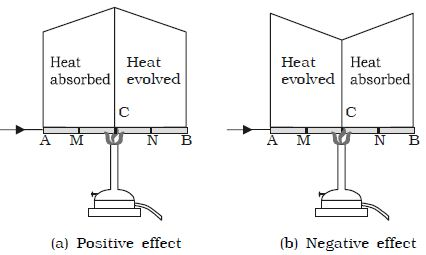# Peltier Effect and Thomson Effect

• If the junctions of a thermocouple are kept at constant temperature and a current is passed through the wires of the thermocouple, it has been found that one of the junction is heated and the other junction is cooled.
• That is heat is produced at one junction (junction which is heated) and absorbed at the other junction (junction which is cooled).
• This effect is known as Peltier effect. This effect is reversible, i.e., when the direction of current is changed, the junction which was heated before gets cooled while the junction which was cooled before gets heated.Cause of Peltier Effect

• When two different metals are joined, contact potential difference is established at the junctions.
• This implies that at the junctions, one metal will be at higher potential than the other.
• For example, in case of Fe-Cu thermocouple, Fe will be at higher potential than Cu.
• Therefore, with the circuit, current flows from Fe to Cu at the junction that is from high potential to low potential.
• So, energy is given out at that junction. Hence, the junction becomes hot.
• Similarly, at another junction, current flows from Cu to Fe, that is from low potential to high potential.
• So, energy is absorbed from that junction. Hence, the junction gets cooled.
• The following table presents the differences between Peltier effect and Joules effect.
•  S.N Peltier Effect Joule’s Effect 1. It is reversible effect. It is irreversible effect. 2. This effect occurs only at the junctions of the dissimilar metals. This effect occurs throughout the body of the conductor. 3. Heat is evolved at one junction and absorbed at the other junction. Heat is not absorbed but is produced throughout the conductor. 4. Heat evolved or absorbed is proportional to current. The heat produced is proportional to the square of the current. 5. The evolution or absorption of heat at a junction depends upon the direction of current.Image source: pininterest The heat produced in a conductor does not depend upon the direction of current.Image source: trendshere

Thomson Effect

• When two ends of the conductor are maintained at different temperatures, an e.m.f is established across it.
• Now, if a steady current is passed through such an unequally heated conductor, heat is absorbed or evolved along the length of the conductor. This effect is called Thomson’s effect.Positive Thomson effect

• In Thomson’s effect, there are some substances in which if current is passed from hot junction to cold junction, heat is evolved.
• When current is passed from the cold junction to the hot junction, heat is absorbed.
• This effect is called positive Thomson effect.
• Substances which show positive Thomson effect are Cu, Ag, Zn, etc.

Negative Thomson Effect

• There are some substances in which if the current is passed from the hot junction to the cold junction, heat is absorbed.
• Similarly, when current is passed from the cold junction to the hot junction, heat is evolved.
• This effect is called negative Thomson effect.
• The substances which show negative Thomson effect are Fe, Pt, Co, etc.

Cause of Thomson Effect

• When two ends of a conductor are maintained at different temperatures, due to the diffusion of free electrons from one end to the other end, the two ends will be at different potential.
• That is, one end will be at higher potential than the other end.
• Now, if steady current is maintained in the conductor in such a way that the current passes from the end at higher potential to the end at lower potential, energy is evolved and heat is generated in the conductor.
• However, if the current passes from the end at lower potential to the end at higher potential, energy is absorbed from the conductor.
• As a result, cooling is observed.
• The following table differentiates Thomson’s Effect and Joule’s Effect.
•  S.N Thomson’s Effect Joule’ Effect 1. It is reversible. It is irreversible. 2. Heat is evolved or absorbed. Heat is always evolved. 3. A temperature difference is required along the length of conductor. No temperature difference is required. 4. It depends upon the direction of current. It is independent of the direction of current. 5. Heat evolved or absorbed is proportional to current. Heat evolved is proportional to the square of the current.

References: